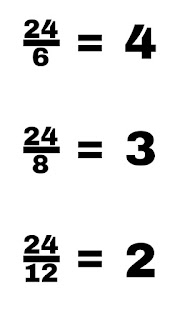## how to find hcf by division method

In this method, the least common divisor is found by dividing the numbers.

Example – Find the least common divisor of 6, 6, 12 using division method.

2|6, 8, 12
2|3, 4, 6
3|3,2,3
0|1,2,1
The least common multiple of 6, 8, 12 = 2ⅹ2ⅹ2ⅹ3 = 24
Note – In this method, the first is divided by small numbers.

#### You may also like

•••